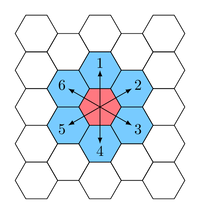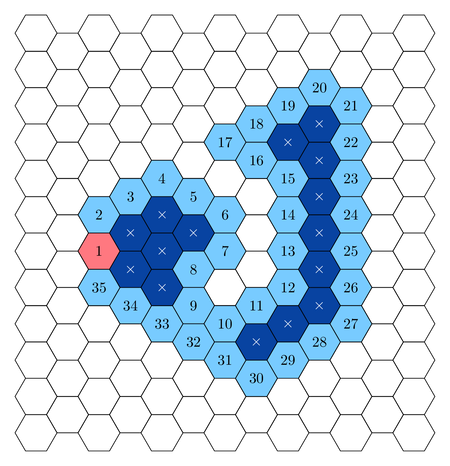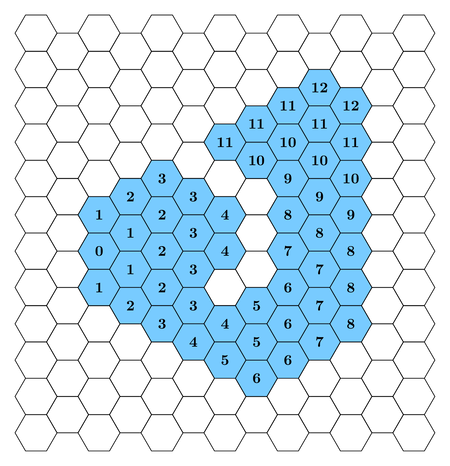# #651. 【APIO2021】六边形领域• 路径是 封闭 的，这意味着在格子序列中，起点格子与终点格子（即初始格子）相同。
• 路径是 简单 的，这意味着在格子序列中，除了初始格子访问过恰好两次（起点和终点分别访问一 次），其他格子只能被访问至多一次。
• 路径是 暴露 的，这意味着在格子序列中，每个格子与至少一个不在序列中出现过的非 内部格子 相邻。
• 如果一个格子不在格子序列中出现过，并且从它出发，在不经过格子序列中任何格子的情况下，（通过若干步移动） 只能访问到有限个格子，我们就称该格子是 内部格子 。

• $1$ 号格子（粉色）是初始格子。
• 被编号的格子（淡蓝色）组成格子序列，编号代表它被访问的顺序。
• 被标上叉号的格子（深蓝色）是内部格子。### 实现细节

int draw_territory(int N, int A, int B, int[] D, int[] L)
• $N$：行动序列中行动的次数。
• $A,B$：分数计算中的常数。
• $D$：大小为 $N$ 的数组，其中 $D[i]$ 表示第 $i$ 次行动的方向。
• $L$：大小为 $N$ 的数组，其中 $L[i]$ 表示第 $i$ 次行动的移动步数。
• 函数应该返回用给出的行动序列所构成的领域中，所有格子的分数总和对 $10^9+7$ 取模后的值。
• 该函数将被调用恰好一次。

### 例子

draw_territory(17, 2, 3,
[1, 2, 3, 4, 5, 4, 3, 2, 1, 6, 2, 3, 4, 5, 6, 6, 1],
[1, 2, 2, 1, 1, 1, 1, 2, 3, 2, 3, 1, 6, 3, 3, 2, 1])



$0$ $1$ $2+0×3=2$ $1×2=2$
$1$ $4$ $2+1×3=5$ $4×5=20$
$2$ $5$ $2+2×3=8$ $5×8=40$
$3$ $6$ $2+3×3=11$ $6×11=66$
$4$ $4$ $2+4×3=14$ $4×14=56$
$5$ $3$ $2+5×3=17$ $3×17=51$
$6$ $4$ $2+6×3=20$ $4×20=80$
$7$ $4$ $2+7×3=23$ $4×23=92$
$8$ $5$ $2+8×3=26$ $5×26=130$
$9$ $3$ $2+9×3=29$ $3×29=87$
$10$ $4$ $2+10×3=32$ $4×32=128$
$11$ $5$ $2+11×3=35$ $5×35=175$
$12$ $2$ $2+12×3=38$ $2×38=76$

### 输入格式

• 第 $1$ 行：$N, A, B$
• 第 $2 + i(0 \le i \le N-1)$ 行：$D[i], L[i]$

### 限制与约定

• $3 \leq N \leq 200\ 000$
• $0 \leq A,B \leq 10^9$
• $1 \leq D[i] \leq 6(0 \leq i \leq N-1)$
• $1 \leq L[i] (0 \leq i \leq N-1)$
• $L$ 中的元素之和不超过 $10^9$。
• 给出的行动序列所对应的路径一定是 封闭、简单暴露 的。

1. ($3$ 分) $N=3,B=0$
2. ($6$ 分) $N=3$
3. ($11$ 分) $L$ 中的元素之和不超过 $2000$
4. ($12$ 分) $B = 0,L$ 中的元素之和不超过 $200\ 000$
5. ($15$ 分) $B = 0$
6. ($19$ 分) $L$ 中的元素之和不超过 $200\ 000$
7. ($18$ 分) $L[i] = L[i + 1] (0 \leq i \leq N-2)$
8. ($16$ 分) 无附加限制